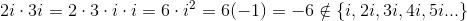SAT II Math II : Mathematical Relationships

Example Questions

Example Question #61 : Mathematical Relationships

Solve: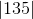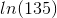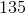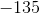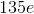Explanation:

The lines on the outside of this problem indicate it is an absolute value problem. When solving with absolute value, remember that it is a measure of displacement from 0, meaning the answer will always be positive.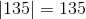For this problem, that gives us a final answer of 135.

Example Question #81 : Sat Subject Test In Math Ii

Solve: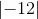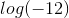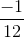Explanation:

The lines on the outside of this problem indicate it is an absolute value problem. When solving with absolute value, remember that it is a measure of displacement from 0, meaning the answer will always be positive.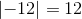For this problem, that gives us a final answer of 12.

Example Question #63 : Mathematical Relationships

Solve: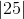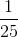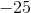Explanation:

The lines on the outside of this problem indicate it is an absolute value problem. When solving with absolute value, remember that it is a measure of displacement from 0, meaning the answer will always be positive.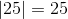For this problem, that gives us a final answer of 25.

Example Question #61 : Mathematical Relationships

What property of arithmetic is demonstrated below?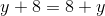Associative

Identity

Commutative

Distributive

Transitive

Commutative

Explanation:

The statement shows that two numbers can be added in either order to achieve the same result. This is the commutative property of addition.

Example Question #62 : Mathematical Relationships

What property of arithmetic is demonstrated below?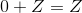Distributive

Identity

Reflexive

Inverse

Transitive

Identity

Explanation:

The fact that 0 can be added to any number to yield the latter number as the sum is the identity property of addition.

Example Question #63 : Mathematical Relationships

What property of arithmetic is demonstrated below?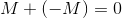Identity

Inverse

Associative

Symmetric

Transitive

Inverse

Explanation:

For every real number, there is a number that can be added to it to yield the sum 0. This is the inverse property of addition.

Example Question #64 : Mathematical Relationships

What property of arithmetic is demonstrated below?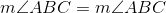Symmetric

Commutative

Symmetric

Reflexive

Transitive

Reflexive

Explanation:

That any number is equal to itself is the reflexive property of equality.

Example Question #65 : Mathematical Relationships

What property of arithmetic is demonstrated below?

If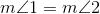then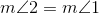.

Commutative

Transitive

Associative

Symmetric

Reflexive

Symmetric

Explanation:

If an equality is true, then it can be correctly stated with the expressions in either order with equal validity. This is the symmetric property of equality.

Example Question #66 : Mathematical Relationships

What property of arithmetic is demonstrated below?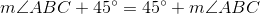Distributive

Identity

Transitive

Associative

Commutative

Commutative

Explanation:

The statement shows that two numbers can be added in either order to yield the same sum. This is the commutative property of addition.

Example Question #7 : Properties And Identities

Which of the following sets is not closed under multiplication?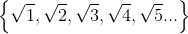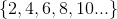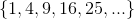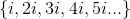All of the sets in the other four responses are closed under multiplication.Explanation:

A set is closed under multiplication if and only the product of any two (not necessarily distinct) elements of that set is itself an element of that set.is closed under multiplication since the product of two even numbers is even: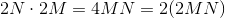is closed under multiplication since the product of two perfect squares is a perfect square: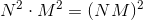is closed under multiplication since the product of two square roots of positive integers is the square root of a positve integer: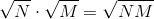But, as can be seen here, is not closed under multiplication: